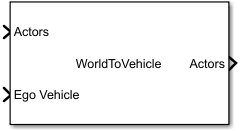# World To Vehicle

Convert actors from world coordinates to ego vehicle coordinates

• Library:
• Automated Driving Toolbox / Driving Scenario and Sensor Modeling

•## Description

The World To Vehicle block converts actor poses from world coordinates to the vehicle coordinates of the input ego vehicle.

## Ports

### Input

expand all

Actor poses in world coordinates, specified as a Simulink bus containing a MATLAB structure.

The structure must contain these fields.

FieldDescriptionType
`NumActors`Number of actorsNonnegative integer
`Time`Current simulation timeReal-valued scalar
`Actors`Actor poses`NumActors`-length array of actor pose structures

Each actor pose structure in `Actors` must contain these fields.

FieldDescription
`ActorID`

Scenario-defined actor identifier, specified as a positive integer.

`Position`

Position of actor, specified as a real-valued vector of the form [x y z]. Units are in meters.

`Velocity`

Velocity (v) of actor in the x- y-, and z-directions, specified as a real-valued vector of the form [vx vy vz]. Units are in meters per second.

`Roll`

Roll angle of actor, specified as a real-valued scalar. Units are in degrees.

`Pitch`

Pitch angle of actor, specified as a real-valued scalar. Units are in degrees.

`Yaw`

Yaw angle of actor, specified as a real-valued scalar. Units are in degrees.

`AngularVelocity`

Angular velocity (ω) of actor in the x-, y-, and z-directions, specified as a real-valued vector of the form [ωx ωy ωz]. Units are in degrees per second.

Ego vehicle pose, specified as a Simulink bus containing a MATLAB structure.

The structure must contain these fields.

FieldDescription
`ActorID`

Scenario-defined actor identifier, specified as a positive integer.

`Position`

Position of actor, specified as a real-valued vector of the form [x y z]. Units are in meters.

`Velocity`

Velocity (v) of actor in the x- y-, and z-directions, specified as a real-valued vector of the form [vx vy vz]. Units are in meters per second.

`Roll`

Roll angle of actor, specified as a real-valued scalar. Units are in degrees.

`Pitch`

Pitch angle of actor, specified as a real-valued scalar. Units are in degrees.

`Yaw`

Yaw angle of actor, specified as a real-valued scalar. Units are in degrees.

`AngularVelocity`

Angular velocity (ω) of actor in the x-, y-, and z-directions, specified as a real-valued vector of the form [ωx ωy ωz]. Units are in degrees per second.

### Output

expand all

Actor poses in vehicle coordinates, returned as a Simulink bus containing a MATLAB structure.

The structure has these fields.

FieldDescriptionType
`NumActors`Number of actorsNonnegative integer
`Time`Current simulation timeReal-valued scalar
`Actors`Actor poses`NumActors`-length array of actor pose structures

Each actor pose structure in `Actors` has these fields.

FieldDescription
`ActorID`

Scenario-defined actor identifier, specified as a positive integer.

`Position`

Position of actor, specified as a real-valued vector of the form [x y z]. Units are in meters.

`Velocity`

Velocity (v) of actor in the x- y-, and z-directions, specified as a real-valued vector of the form [vx vy vz]. Units are in meters per second.

`Roll`

Roll angle of actor, specified as a real-valued scalar. Units are in degrees.

`Pitch`

Pitch angle of actor, specified as a real-valued scalar. Units are in degrees.

`Yaw`

Yaw angle of actor, specified as a real-valued scalar. Units are in degrees.

`AngularVelocity`

Angular velocity (ω) of actor in the x-, y-, and z-directions, specified as a real-valued vector of the form [ωx ωy ωz]. Units are in degrees per second.

## Parameters

expand all

Source of the name for the actor poses bus returned in the Actors output port, specified as one of these options:

• `Auto` — The block automatically creates an actor poses bus name.

• `Property` — Specify the actor poses bus name by using the Actors bus name parameter.

Name of the actor poses bus returned in the Actors output port, specified as a valid bus name.

#### Dependencies

To enable this parameter, set Source of actors bus name to `Property`.

• `Interpreted execution` — Simulate the model using the MATLAB interpreter. This option shortens startup time. In ```Interpreted execution``` mode, you can debug the source code of the block.

• `Code generation` — Simulate the model using generated C/C++ code. The first time you run a simulation, Simulink generates C/C++ code for the block. The C code is reused for subsequent simulations as long as the model does not change. This option requires additional startup time.

## Version History

Introduced in R2020a